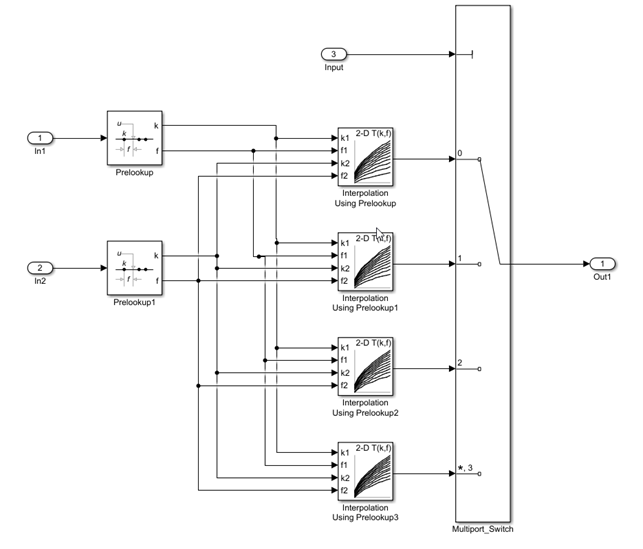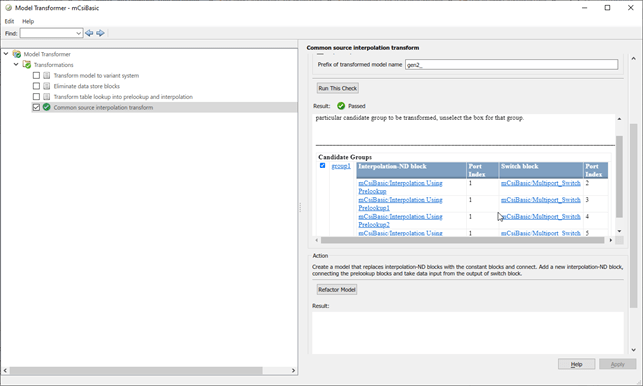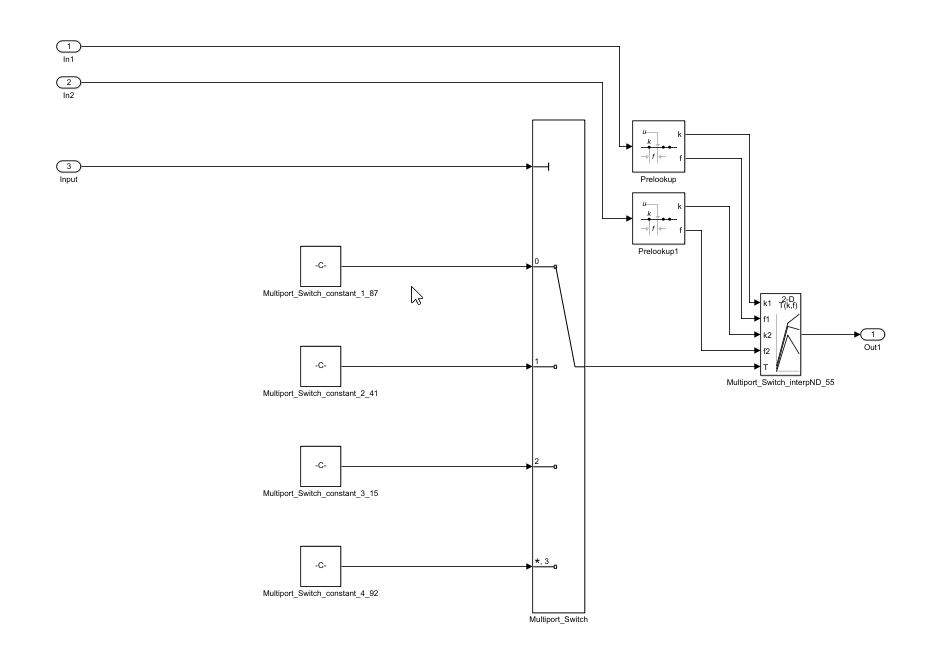## Improve Code Efficiency by Merging Multiple Interpolation Using Prelookup Blocks

You can use the Model Transformer tool to refactor a modeling pattern to improve the efficiency of generated code. The Model Transformer identifies and merges multiple Interpolation Using Prelookup blocks that have same input signals connected from the outputs of Prelookup blocks into a single Interpolation Using Prelookup block.

The Model Transformer works if the properties of Interpolation Using Prelookup blocks are same except for Table data. Reducing the number of Interpolation Using Prelookup blocks in a model reduces the number of variable assignments in the code, which improves the efficiency of the generated code. You can use the Model Transformer app or programmatic commands to refactor the model.

The Model Transformer can replace multiple Interpolation Using Prelookup that:

• Have the same input signals connected to Prelookup blocks with the same index and fraction parameters

• Have the output signals connected to the same Multiport Switch block

• Have the same breakpoint specification, values, and data types

• Have the same algorithm parameters

• Have the same data type for fraction parameters

### Merge Interpolation Using Prelookup Blocks Using the Model Transformer App

This example shows how to use the Model Transformer to identify redundant Interpolation Using Prelookup blocks, and then refactor the model.The model `ex_interpolation_optimize` uses Prelookup blocks to input signals to several Interpolation Using Prelookup blocks. The output of these Interpolation blocks are connected to a Multiport Switch block.

In this example, you identify Interpolation Using Prelookup blocks that qualify for transformation and replace them with a single Interpolation Using Prelookup block and Constant blocks connected to Multiport Switch block.

1. Save the model to your working folder.

2. On the Apps tab, click Model Transformer.

3. In the Transformations folder, select the Replace Interpolation Using Prelookup Blocks check.

4. Select the Skip Interpolation-ND blocks in libraries from this transformation option to avoid replacing Interpolation Using Prelookup blocks that are linked to a library.

5. In the Prefix of transformed model field, specify a prefix for the refactored model.

6. Click Run This Check. The top Result table contains hyperlinks to the Interpolation Using Prelookup blocks and the corresponding Multiport Switch block port indices.

7. Clear the check boxes under Candidate Groups for the groups that you do not want to transform.

8. Click Refactor Model. The Result table contains a hyperlink to the new model. The table contains hyperlinks to the shared Interpolation Using Prelookup blocks and corresponding Multiport Switch block ports. The tool also creates an `m2m_ex_interpolation_optimize` folder that contains the new `gen_ex_interpolation_optimize.slx` model.The two Prelookup blocks Prelookup and Prelookup1 in the `gen_ex_interpolation_optimize.slx` model connect to the single Interpolation Using Prelookup block and the Constant blocks are connected to the Multiport Switch block port to give the Table data as input.### Merge Interpolation Using Prelookup Blocks Programmatically

To use the Model Transformer programmatically, use:

• `Simulink.ModelTransform.CommonSourceInterpolation.identifyCandidates` to identify candidates for transformation

• `Simulink.ModelTransform.CommonSourceInterpolation.refactorModel` to refactor the model

1. Save the model `ex_interpolation_optimize` in the current working directory.

2. To identify candidates qualified for transformation, use the function `Simulink.ModelTransform.CommonSourceInterpolation.identifyCandidates` to create the object `transformResults`.

```transformResults = Simulink.ModelTransform.CommonSourceInterpolation.identifyCandidates('ex_interpolation_optimize') ```
```transformResults = Results with properties: Candidates: [1×1 struct]```

The `transformResults` object has one property, `Candidates`, that is a structure with two fields, `InterpolationPorts` and `SwitchPorts`.

``` transformResults.Candidates = struct with fields: InterpolationPorts: [4×1 struct] SwitchPorts: [4×1 struct]```

3. View the `InterpolationPorts` field.

`transformResults.Candidates.InterpolationPorts = `
``` 4×1 struct array with fields: Block Port```

The `InterpolationPorts` field consists of two arrays, `Block` and `Port`. Similarly, the `SwitchPorts` has the same properties.

4. Convert the `Candidates.InterpolationPorts` and `Candidates.SwitchPorts` fields to tables.

```struct2table(transformResults.Candidates.InterpolationPorts) struct2table(transformResults.Candidates.SwitchPorts)```
```ans = 4×2 table Block Port _____________________________________________ ____ {'ex_interpolation_optimize/Interpolation↵Using Prelookup' } 0 {'ex_interpolation_optimize/Interpolation↵Using Prelookup1'} 0 {'ex_interpolation_optimize/Interpolation↵Using Prelookup2'} 0 {'ex_interpolation_optimize/Interpolation↵Using Prelookup3'} 0 ans = 4×2 table Block Port ______________________________ ____ {'ex_interpolation_optimize/Multiport_Switch'} 1 {'ex_interpolation_optimize/Multiport_Switch'} 2 {'ex_interpolation_optimize/Multiport_Switch'} 3 {'ex_interpolation_optimize/Multiport_Switch'} 4 ```

Use the `SwitchPorts` to see which Interpolation Using Prelookup blocks are connected to which Multiport Switch block port.

5. To refactor the model, use the function `Simulink.ModelTransform.CommonSourceInterpolation.refactorModel`. This function uses the object `transformResults` from `identifyCandidate` function.

```refactorResults = Simulink.ModelTransform.CommonSourceInterpolation.refactorModel(transformResults) ```
```refactorResults = RefactorResults with properties: ModelName: 'ex_interpolation_optimize' ModelDirectory: '' TraceabilityInfo: [4×1 containers.Map]```

The `ModelName` and `ModelDirectory` properties of the `refactorResults` object list the name and location of the refactored model. `TraceabilityInfo` is a `containers.Map` object that lists the block tracing information.

### Conditions and Limitations

The Model Transformer cannot replace Interpolation Using Prelookup blocks if:

• The Interpolation Using Prelookup blocks are in commented-out regions or inactive variants.

• The Interpolation Using Prelookup blocks are masked.

• The Model Transformer app does not replace Interpolation Using Prelookup blocks across the boundaries of atomic subsystems, referenced models, or library-linked blocks.